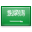# Range (mathematics)${displaystyle f}$is a function from domain X to codomain Y. The yellow oval inside Y is the image of ${displaystyle f}$. Sometimes "range" refers to the image and sometimes to the codomain.

In mathematics, and more specifically in naive set theory, the range of a function refers to either the codomain or the image of the function, depending upon usage. Modern usage almost always uses range to mean image.

The codomain of a function is some arbitrary super-set of image. In real analysis, it is the real numbers. In complex analysis, it is the complex numbers.

The image of a function is the set of all outputs of the function. The image is always a subset of the codomain.

## Distinguishing between the two uses

As the term "range" can have different meanings, it is considered a good practice to define it the first time it is used in a textbook or article.

Older books, when they use the word "range", tend to use it to mean what is now called the codomain. More modern books, if they use the word "range" at all, generally use it to mean what is now called the image. To avoid any confusion, a number of modern books don't use the word "range" at all.

As an example of the two different usages, consider the function ${displaystyle f(x)=x^{2}}$as it is used in real analysis, that is, as a function that inputs a real number and outputs its square. In this case, its codomain is the set of real numbers ${displaystyle mathbb {R} }$, but its image is the set of non-negative real numbers ${displaystyle mathbb {R} ^{+}}$, since ${displaystyle x^{2}}$is never negative if ${displaystyle x}$is real. For this function, if we use "range" to mean codomain, it refers to ${displaystyle mathbb {displaystyle mathbb {R} ^{}} }$. When we use "range" to mean image, it refers to ${displaystyle mathbb {R} ^{+}}$.

As an example where the range equals the codomain, consider the function ${displaystyle f(x)=2x}$, which inputs a real number and outputs its double. For this function, the codomain and the image are the same (the function is a surjection), so the word range is unambiguous; it is the set of all real numbers.

## Formal definition

When "range" is used to mean "codomain", the image of a function f is already implicitly defined. It is (by definition of image) the (maybe trivial) subset of the "range" which equals {y | there exists an x in the domain of f such that y = f(x)}.

When "range" is used to mean "image", the range of a function f is by definition {y | there exists an x in the domain of f such that y = f(x)}. In this case, the codomain of f must not be specified, because any codomain which contains this image as a (maybe trivial) subset will work.

In both cases, image f ⊆ range f ⊆ codomain f, with at least one of the containments being equality.

### أهلاً ومرحباً بكم## قسم الرياضيات### التوقيت والتقويم

```_time.start(["thetimenow"]);
```

```
```

```

```

### محرك بحث جوجل```

```

### للتواصل1. الهاتف : 0164044771

تحويلة: 4771

(QR Code)

mailto:mm.mousa@mu.edu.sa### إعلانات1- الاختبار الفصلى الثانى لمقرر التحليل العددى (يوم الاحد الموافق 3 / 7/ 1440 هـ)

2- الاختبار الفصلى الثانى لمقررحساب المتجهات (يوم الثلاثاء الموافق 5 / 7 / 1440 هـ)

### الساعات المكتبيةالأثنين: 10 - 12

الثلاثاء: 8 - 10

الأربعاء: 8 - 10

### بعض الخدمات الإلكترونية لعمادة تقنية المعلومات### آلة حاسبة

```

```

### التقويم الجامعى### بعض الجوائز والتكريمات### إحصائية الموقع

عدد الصفحات: 258

البحوث والمحاضرات: 155

الزيارات: 101562